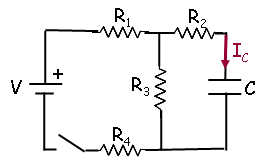# Problem: A circuit is constructed with four resistors, one capacitor, one battery and a switch as shown. The values for the resistors are: R1 = R2 = 36 Ω, R3 = 77 Ω and R4 = 120 Ω. The capacitance is C = 67 μF and the battery voltage is V = 12 V. The positive terminal of the battery is indicated with a + sign.A. The switch has been open for a long time when at time t = 0, the switch is closed. What is I4(0), the magnitude of the current through the resistor R4 just after the switch is closed?B. What is Q(∞), the charge on the capacitor after the switch has been closed for a very long time?C. After the switch has been closed for a very long time, it is then opened. What is Q(topen), the charge on the capacitor at a time topen = 555 µs after the switch was opened?D. What is IC,max(closed), the current that flows through the capacitor whose magnitude is maximum during the time when the switch is closed? A positive value for the current is defined to be in the direction of the arrow shown.E. What is IC,max(open), the current that flows through the capacitor whose magnitude is maximum during the time when the switch is open? A positive value for the current is defined to be in the direction of the arrow shown.

###### FREE Expert Solution

Equivalent resistance for 2 resistors in parallel:

$\overline{){{\mathbf{R}}}_{{\mathbf{eq}}}{\mathbf{=}}\frac{{\mathbf{R}}_{\mathbf{1}}{\mathbf{R}}_{\mathbf{2}}}{{\mathbf{R}}_{\mathbf{1}}\mathbf{+}{\mathbf{R}}_{\mathbf{2}}}}$

Equivalent resistance for resistors in series:

$\overline{){{\mathbf{R}}}_{{\mathbf{eq}}}{\mathbf{=}}{{\mathbf{R}}}_{{\mathbf{1}}}{\mathbf{+}}{{\mathbf{R}}}_{{\mathbf{2}}}{\mathbf{+}}{\mathbf{.}}{\mathbf{.}}{\mathbf{.}}{\mathbf{+}}{{\mathbf{R}}}_{{\mathbf{n}}}}$

Ohm's law:

$\overline{){\mathbit{i}}{\mathbf{=}}\frac{\mathbf{V}}{\mathbf{R}}}$

A.

Req = R1 + (R2)(R3)/(R2 + R3) + R4

Req = 36 + (36)(77)/(36 + 77) + 120 = 180.53Ω

From Ohm's law:

85% (182 ratings)###### Problem Details

A circuit is constructed with four resistors, one capacitor, one battery and a switch as shown. The values for the resistors are: R1 = R2 = 36 Ω, R3 = 77 Ω and R4 = 120 Ω. The capacitance is C = 67 μF and the battery voltage is V = 12 V. The positive terminal of the battery is indicated with a + sign.A. The switch has been open for a long time when at time t = 0, the switch is closed. What is I4(0), the magnitude of the current through the resistor R4 just after the switch is closed?

B. What is Q(∞), the charge on the capacitor after the switch has been closed for a very long time?

C. After the switch has been closed for a very long time, it is then opened. What is Q(topen), the charge on the capacitor at a time topen = 555 µs after the switch was opened?

D. What is IC,max(closed), the current that flows through the capacitor whose magnitude is maximum during the time when the switch is closed? A positive value for the current is defined to be in the direction of the arrow shown.

E. What is IC,max(open), the current that flows through the capacitor whose magnitude is maximum during the time when the switch is open? A positive value for the current is defined to be in the direction of the arrow shown.